## (for dummies)

This is an attempt at clarifying the meaning of words like Volt and Watt, and giving you simple ways to figure out what you should and should not do with electricity.

# What this is Not

What we’re not going to do here:  talk about the physics of electricity, explain how batteries work, how power stations make electricity, worry about chemistry.

# What is it?

The words “electricity” and “electric” are vague, they refer to a large number of phenomena.  Electricity is used for lighting, heating, cooling, running the motors of household appliances, in medecine and so on.

It is also used in communication:  radio, TV, phones, the internet, computers.  In communication however there is another component at work:  encoded information.  Applications of this type belong to electronics and we will not consider them here, though they do use the same electrical phenomena.

Broadly speaking

electricity is about power

while

electronics is about information.

# Example 1

Let us start with looking at a familiar example:  a small pocket torch.  It has a LED bulb to emit the light, a switch to switch it on and off, and it is powered by a battery:

When the switch is pressed and contact is made, something called electric current flows from the positive tip of the battery, through the bottom of the bulb, along the wires holding the LED, through the LED where it causes the LED to emit light, back through the side of the bulb, through the switch and finally back into the battery.

Like a current of water is composed of drops of water, electric current is composed of electric charges.

Your pocket torch may be different in some unimportant aspects: the switch may sit before the current goes into the bulb, the bulb may be a classic incandescent filament bulb, and so on.

The important arrangement is that there is a source of electric power (the battery) and a consumer of that power (the bulb) all connected by an uninterrupted (closed) circuit of metal parts (usually wires).

We can present this arrangement in a simplified diagram:

The circle with the arrow represents the power source, the arrow indicates the direction in which the source pushes the current;  the grey rectangle represents the consumer, usually called the load, and the orange lines are the wires completing the circuit.

The current flow is always from the top of the power source (tip of the arrow), through the wires and through the load, back to the bottom of the power source.

How do we know there is a flow of something?  Could it not be something else that is happening?  Perhaps just making the contact lights the bulb and there is nothing whatever moving around?  We do know that electric charges are actually flowing in the wires:  although we can’t see them, there are some electric effects (lightning, electro-plating, cathode rays) that show a flow of electrically charged particles.  The particles are usually electrons.  However, this detail does not concern us here;  all we need to remember is that electric charge is moving through the circuit.

# Tension — Current — Resistance

Look again at the battery powered LED torch.

When the torch is on, electric charge flows around the circuit:  the more charge per second that goes around, the more intense the electric current is.  Electric current is measured in charge per second, just like the rate of fluid flow in a pipe would be measured in litres per second.

The LED bulb does not let the current through freely, the battery needs to push it through.  The force with which the battery pushes is called its electric tension or more commonly voltage.  The bulb has some resistance to the flow of current:  the amount of current that passes is an equilibrium act between the force of the voltage and the amount of resistance of the bulb.

We need some units to talk about electricity, in gthe same way that we need units of length, time and mass (metres, seconds and kilograms) to talk about things happening in mechanics.

Here are important definitions of electric units:

• Charge is measured in Coulomb (and we won’t use this unit often).  A Coulomb is just an agreed, fixed number of electrons.
• Current is measured in Coulomb per second, it’s like a flow.
• Tension or voltage is measured in Volt.  It’s very much like a force.
• Resistance is measured in Ohm.  This is a more difficult concept.

## Probable confusion

It’s easy to grasp that the flow of the charge is measured in Coulomb per second.

Unfortunately nobody uses “Coulomb per second”, instead people say “Ampère”.  One Ampère is just a single word for the phrase “one Coulomb per second”.  If later on you feel you are getting confused, just replace Ampère with Coulomb per second.

## Notation

It’s a bit tedious to use words or phrases when doing calculations.  In mechanics we use the single letter l for length, measuring it in m (for metres); we use t for time, measuring it in s (for seconds), and so on.

Similarly, in electricity we use:

• I for intensity of current, measured in Ampère = A
• U for tension (don’t ask why) measured in Volt = V
• R for resistance, measured in Ohm = Ω

We can now label our basic circuit:

# First Law

In the simple, labelled circuit the current I is limited by the resistance R of the load and by the voltage U of the power source.

We would not be surprised if the current increased if we increased the voltage:  push harder, more will flow.

Likewise we would not be surprised if the current diminished if we increased the resistance of the load.

In fact there is a precise and simple relation.  If we double the voltage then the current doubles.  If we double the resistance then the current halves.  This just means that the current is proportional to the voltage and inversely proportional to the resistance:

$I=\frac{U}{R}$

This is easy to remember.  The current is the result of hooking the load to the source, it’s what we want to calculate:  it is what we need a formula for.  If the voltage increases the current increases, so the U is on top; if the resistance is bigger then the current is lower so the R is below.

Again:  if you forgot how to start writing this formula, always remember that it’s the current that is the result of putting a power source and a load into a circuit, so it is the current we are normally computing.

The relationship $I=\frac{U}{R}$ is more commonly known as Ohm’s law.

Current is the result of voltage pushing charge through resistance.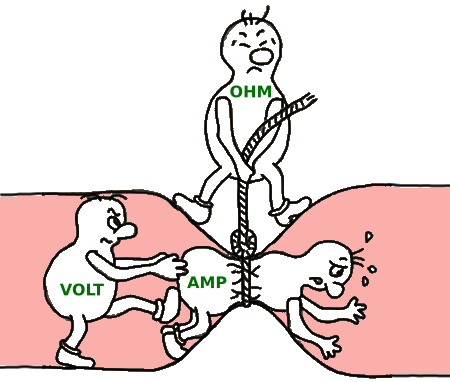humouristically…

(I do not know the author of this cartoon, if there is any problem reproducing it here she/he can contact me by e-mail: robert at cailliau org)

## Other forms of Ohm's Law

The relation between current, voltage and resistance can be used to calculate any one of those three if the other two are known (remember your hgih school maths).  We have three formulae, all equivalent:

Find the current:  $I=\frac{U}{R}$

Find the resistance:  $R=\frac{U}{I}$

Find the voltage:  $U=I×R$

# Power and Energy

## Power

The load in an electric circuit is used for some purpose:  it is a light or an electric motor turning something, or an oven heating up food.  When the load is active, energy flows from the power source to the load.  The load uses up energy.  How do we count this?

Without going into too much detail, we understand that at a given voltage more energy flows if the current increases, and also more energy flows if the current remains the same but the voltage is higher.

It turns out there is a very simple relationship here too.  The energy flow is proportional to both the voltage and the current:

$P=U×I$

This quantity is called power; its symbol is P and its unit is V×A.  You can sometimes find VA mentioned on power adapters.

The more commonly used unit is W (Watt) instead of the combination VA (in certain circumstances there are good reasons for using VA instead of W, but we won’t go into that here).

We can now add a line to our list of electric quantities:

• I for intensity of current, measured in Ampère = A
• U for tension (don’t ask why) measured in Volt = V
• R for resistance, measured in Ohm = Ω
• P for power, measured in Watt = W

You will of course have seen labels on electric devices that tell you some amount of W:  the device’s power rating.  The higher this number, the more energy per second it uses.

Similar to Ohm's law, we can use the relationship to calculate any one of the three quantities if the other two are known:

Find the current:  $I=\frac{P}{U}$

Find the voltage:  $U=\frac{P}{I}$

Find the power:  $P=U×I$

## Energy

Power is not energy, it is amount of energy per second.

The letter used for energy is E, and the measurement unit is Joule, or J.

You will sometimes see an old-style unit which should no longer be used:  the calorie.  A calorie is about 4.184 Joules.  A calorie on food labels is actually a kilo-calorie or 1000 calories.

## Another probable confusion…

So power, which is flow of energy, is really measured in Joules per second or J/s.

But nobody says “Joules per second”, people say “Watt”.  One Watt is just short for the phrase “one Joule per second”.  And again, if later on you feel you are getting confused, just replace Watt with Joules per second and things may become clearer.

# Exercises

Time for some exercises.  Look at this label on a toaster: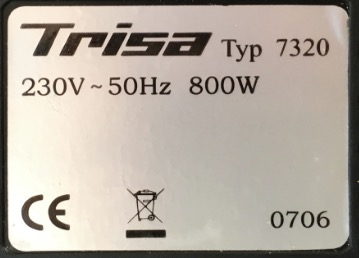Label on a toaster

The important things for us here are:  230V ~50Hz 800W

This means it is made to operate on an electric supply of 230V, alternating current at 50Hz, and that when it operates it will use 800W (800 Joules of energy per second).

That it is for alternating current is of no concern here, and that the current alternates 50 times per second is also of no concern.

We know that P=U×I and here we are told that P=800W with U=230V.  Then what will the current be?  We remember that if P=U×I then also I=P/U, so we get

I=800W/230V=3.48A

About 3.5 ampère.

Now we look at the kettle: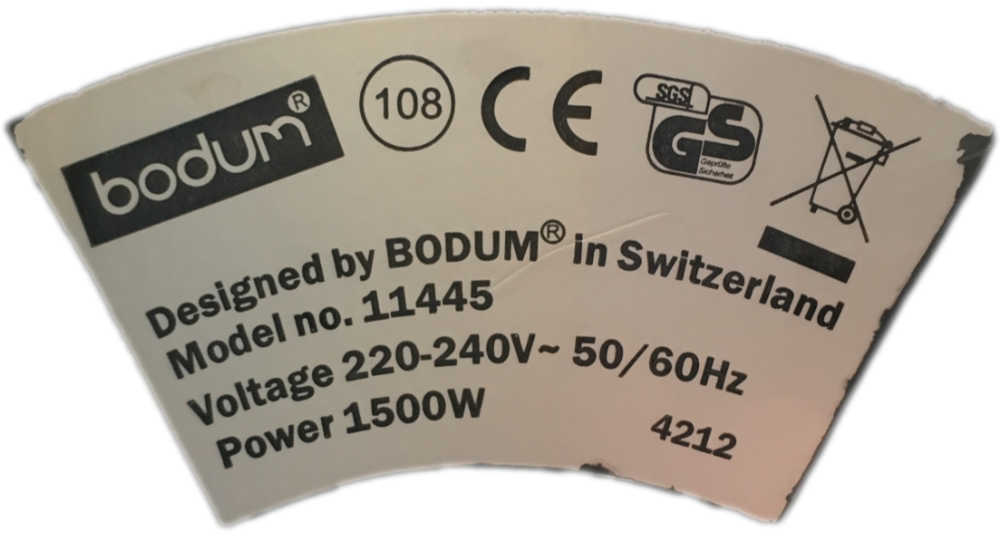Label on a kettle

This device uses 1500W at 230V, so the current it draws when boiling water is I=1500W/230V = 6.52A

If we operate both at the same time from the same socket (perhaps using a power strip), we will draw a total of 6.52A+3.5A=10.02A of current.

The resistance of the toaster is R=U/I=230V/3.5A=65.7Ω

The resistance of the kettle is 230V/6.52A=35.3Ω

And that is also what we would expect:  the toaster resists electric current flow about twice as much as the kettle, and therefore it uses about half the power.

Finally we look at the back of the TV: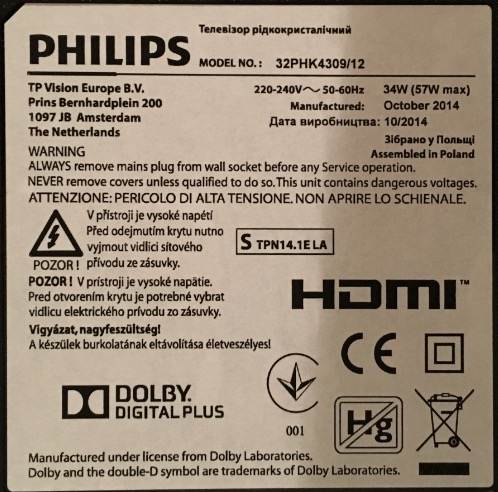Label on a TV

A bit more difficult to find, but it does read: 220V-240V ~50-60Hz 34W(57W max).  Why are there two power ratings?  Because the power consumption depends on how bright you make the screen and how loud you play the speakers.  Let's use the maximum:

The TV draws 57W/230V=0.25A.  Its resistance should be very high if it lets through only so little current:  230V/0.25A=920Ω.  Yes, indeed.

People with a deeper knowledge of electricity will have become very uneasy by now:  I have glossed over a lot of things such as the difference between alternating and direct current, and worse, I have used the term resistance for a device like a TV which is definitely not a resistor in the electronics or even electric sense.  But it does resist the current flow, and it is useful to have an idea of the magnitude with which it does.

By the way, direct current and alternating current are misnomers:  it's the tension that is direct or alternating.  The current is always a result of what load is put in the circuit.  A house socket has alternating voltage on it, even if there is nothing connected to it and no current flows at all.  If you connect a diode before the load, then the current is no longer alternating but the voltage still is.

# Real Confusion

It is very important to make a distinction between power and energy.

When we say a tool is powerful, we mean it is capable of delivering a large flow of energy.  A hot water kettle of 2000W is more powerful than one of 1000W.

Because many devices are rated in thousands of W, the rating is often in kiloWatt or kW.  1kW = 1000W.  Other multiples are MegaWatt or MW, 1MW=1’000’000W and GigaWatt or GW, 1GW=1’000’000’000W.

A few examples, not all electric though:

power rating for some devices
deviceratingrating in single Wnote
LED lamp giving 600 lumen of light 6W 6  W so count about 100 lumen light output per W
laptop computer 40W 40  W
normal incandescent lamp giving 600 lumen of light 60W 60  W now being replaced by LEDs
human, sleeping 50W 50  W
human, at work 100W 100  W
farm labourer 150W 150  W when working in the fields
large TV 200W 200  W
1m2 solar panel 275W 275  W at very optimal circumstances during the day
vacuum cleaner 800W 800  W older ones can be above 1kW but more power does not necessarily mean they work better
average home 1kW 1'000  W averaged over a year
hot water kettle 1500W 1'500  W
oven 2kW 2'000  W
small car 50kW 50'000  W that's when it runs at maximum power
“Jet d'Eau” fountain of Geneva 1MW 1'000'000  W or 1000kW
wind turbine 5MW 5'000'000  W that is of course production, not consumption
small nuclear power station 1GW 1'000'000'000  W or 200 wind turbines at full power day and night

That table gives flow of energy, Joules per second.  To find how much energy has been used, we need to know how long the device has been operating.

Say you switch the LED lamp on for one second:  it will use 6 Joules per second, and do that for one second, so it has used up 6 Joules.  Now leave it on for a minute:  that needs 60×6 or 360 Joules.

Now take the hot water kettle of 1500W.  If it is filled with water and takes 10 minutes to bring it to boil, it will have spent 1500 Joules per second during 600 seconds, or 1500×600=900'000 Joules.

So far so good.  Let the oven bake something for three hours.  It will have used 2000 Joules per second for 3×3600=10'800 seconds, so it will have spent 2000×10'800=21'600'000 Joules of energy to bake whatever was placed in it.

You will have to pay the electricity company for all that energy.  How much does a Joule cost?  It is a fairly small unit if just baking something will need millions.  We need a bigger unit. We could use a mega-Joule, one million Joules, or 1MJ.  The baking would then have taken 21.6MJ.  If a MJ costs say 3 cents then we would have to pay 21.6×3 = 64.8 cents.
That would be an easy calculation, but unfortunately that's not how it was done historically.  Instead, we started to go by the amount of energy used over an hour by a device that has a power of 1kW, and we called that a kiloWatthour or kWh.

The oven used 2kW during 3 hours, or 2kWx3h=6kWh.  Obviously that is not a metric unit (the hour is not the metric unit of time).

How much is a kWh?  it is 1000W during one hour (3600 seconds).  It is 1000J/s×3600s = 3'600'000 Joules, or 3.6MJ.  And it costs about 13 cents or 3.6 cents per MJ.  The baking cost 21.6MJ×3.6=77.7 cents.

Now obviously it is quicker to estimate electric energy consumption by the hour than by the second:  just multiply the power of the device in kW by the number of hours it has been going and you find the number of kWh of energy to be paid for.

## Flow versus quantity

The flow of electric charge is measured in Ampères, or Coulomb per second.  The quantity of charge is measured in Coulomb.

The flow of electric energy is electric power, it is measured in Watt, or Joules per second.  The quantity of energy is measured in Joules.

More powerful devices will have energy flow measured in kW, or kiloWatt, one thousand Watt.  The amount of energy they used will be measured by the hour of usage, not by the second, and the unit is then the kWh.

Do not let this confuse you:  a kW is power, a kWh is an amount of energy.

Here are a few sentences I sometimes hear:

“My bill this month was for 785 kilowatt.”  Wrong statement.  Your bill was for 785 kilowatthours.

“This clothes dryer uses only 1kW” — “Is that 1 kW per hour?”  Wrong question:  it is indeed 1kW, which is 1000 Joules per second.  Or, it uses 1 kilowatthour per hour.

# Real Devices

In reality, the components in a basic circuit are not perfect.  The wires connecting the power source to the load are not without resistance themselves.

The power source also has some internal resistance against the flow of its own charge.

If the resistance is very low and therefore a very large current flows, the source may not be able to keep up and its voltage drops.

If the load is very large, the voltage of the power source may not be large enough to make enough current flow to make the load work:  you can’t start a car engine with a single AA battery.

If a large current is needed and the wires connecting the source to the load are too thin to carry it, then their own resistance becomes important, they heat up and may melt.  See about overcurrent down below.

However, in normal everyday circumstances, Ohm's law $I=\frac{U}{R}$ applies well.

# Real Life

Where do you see volts, amperes and ohms in real life?  Sometimes they can be found on a product's identification label, as we saw in the exercises.

## Volts

The voltage of batteries is printed on them:  AA and AAA format batteries have 1.5V, lithium button batteries also are 1.5V.  Small rectangular batteries are 9V.  Car batteries are 12V or 24V.

Note that these batteries, rechargeable or not, are based on chemical processes.  Although the physics is similar for different types, the voltages are not exactly the same:  lithium batteries do not give exactly the same voltage as alkaline batteries, but the general rule is that it is very close to 1.5V or a whole multiple thereof (3, 4.5, 6, 9, …) .

Batteries built into devices such as smartphones, cameras, portable tools etc. have different voltages, and they are usually printed on the device.

USB sockets deliver data but also power, and the power is standardised at 5V

House wall sockets deliver 240V in most of the world.

## Ampères

Most devices do not have a constant rate of current flow:  it depends on how they are used.  A smartphone may use a high current when a lot of apps are active at the same time, and very little when it is in standby mode.  Therefore most devices do not have their current printed on their product label.

However, you will find current values on chargers:  a smartphone charger with a USB socket will usually allow a maximum current of 2A to flow.  This is a useful indication but it is a maximum, not what really flows because that depends on the device connected to it.  If a device’s battery presents a small resistance during charging, then the current from the charger may have to be higher and if the charger cannot deliver it, charging will be slow or may even fail.

Some power strips and multi-socket adapters give an allowed maximum current, which is usually around 10A.

## Ohms

You will almost never see Ohms.  I cannot think of any household example in fact.

# Overcurrent

Connecting wires between power source and load have their own resistance to electric current flow.  Although in most practical cases this resistance can be neglected, it does cause wires to heat up if the current gets to be very high.  That is a frequent cause of electric fires, so devices have been invented to shut of a circuit when overcurrent is detected.

## Protection against overcurrent

House electric sockets are connected by copper wires all the way back to the main electric distribution box placed somewhere in the house by the utility company.  This box is usually in some utility room (garage, laundry room, workshop, …)

The wires may be quite long.  If you plug a device into some socket and the device draws a lot of current then those wires may warm up because of their own resistance and in extreme cases may cause a fire.  For that reason the current through the wires is limited by a circuit breaker.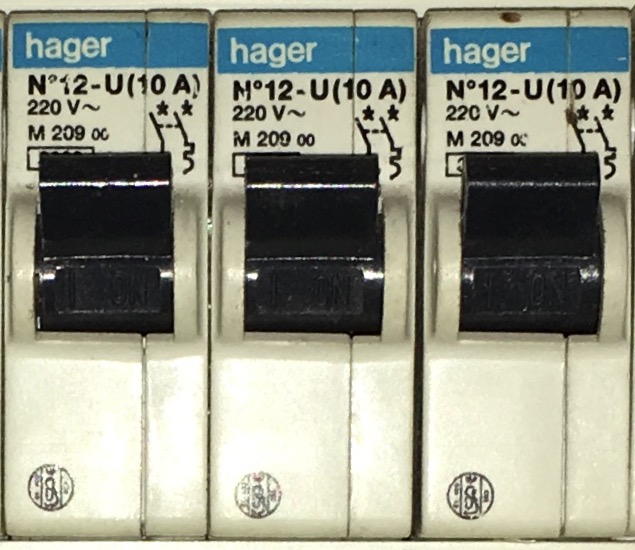Circuit breakers

The early circuit breakers were actual fuses, i.e. they physically melted when the current through them went over a certain limit.  A fuse had to be replaced by a new one once it had melted.  Today all circuit breakers are electromechanical devices that “trip”:  they switch the circuit off when the current becomes too high.  They can be reset again.

Circuit breakers trip when the current in the circuit exceeds a certain value. This value is marked on the breaker.  The three shown in the picture are all marked “(10A)”

### Dealing with Overcurrent

The two most common causes of overcurrent are:

• accidental short circuit
• too many devices on the same socket

Short circuits are caused by faulty wiring or some failure inside a device, so that the current can bypass the load and return to the source immediately.  Because the current no longer encounters any resistance from the load, the only resistance remaining is that of the wires to the socket, they will heat up but the overcurrent protection will switch the circuit off.

Obviously, resetting the circuit breaker will do no good:  you have to first unplug the faulty device or remove whatever the cause of the short circuit is.

Too many devices on the same socket usually happens when a power strip (or multi-socket adapter) is connected to a socket and multiple devices are plugged into the strip. But you can easily calculate how far you can go.  For example, one of the circuit breakers in the image above will trip at 10A. At a voltage of 220V that is a total power of P=220×10=2200W.  You can now add all the power ratings of all the devices that are plugged into sockets protected by that circuit breaker.  Say there is nothing connected except for one socket with a power strip, and into that are plugged a laptop power supply of 50W, a battery charger of 10W, a fan of 100W, a 300W lamp lighting the ceiling, and a TV of 120W. The total is 50+10+100+300+120=580W. That is a fair number of devices, but the total power used is still way below the allowance.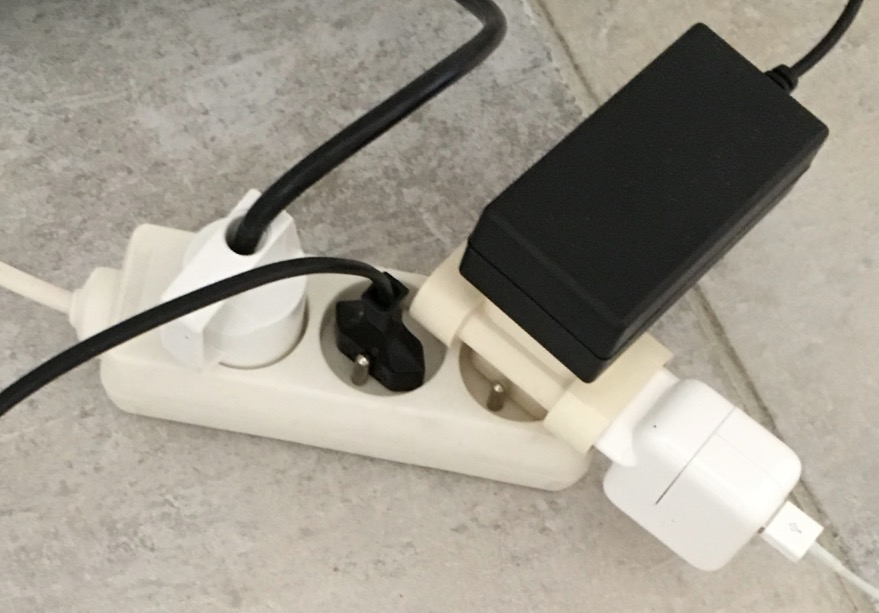a seemingliy overloaded power strip

However, should you unplug the power strip from the wall socket and plug a single 2500W electric radiator into it, you would instantaneously trip the breaker.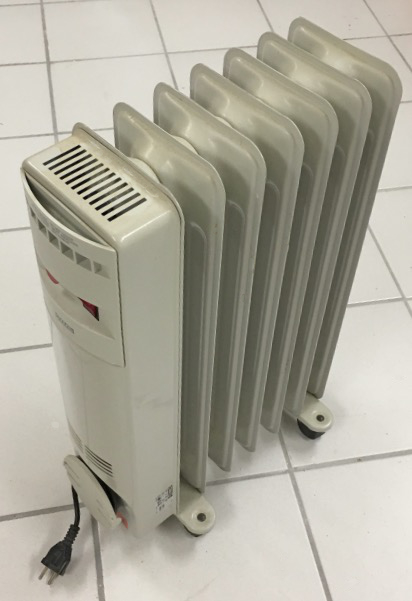electric radiator

It is not the number of devices that is important, but the total energy flow, the sum of their power ratings.

# Possible Confusions

It is useful to repeat here the concepts that may lead to potential confusions.  Only the correct notions are stated.

an Ampère is a flow, it is a Coulomb of electric charge per second.

a Watt is power, it is a flow, it is a Joule of energy per second.

a kW is power, it is a flow of 1000 Joules per second.

a kWh is energy, it is the quantity of energy used by a one-kW device over the period of one hour.  It corresponds to 3'600'000 Joules.

## The final straw…

You will see batteries and rechargeable battery packs marked with their energy content in mAh.  That is milli-Ampère-hours.  This makes NO SENSE:  mAh is a measure of charge, not energy.

An Ampère is a Coulomb per second, a flow of charge.  During one hour, a current of 1A has given 3600 Coulombs of charge.  They went from the positive to the negative terminal of the power source, spending energy in some load.  A milli-Ampère-hour is one thousandth of that, or 3.6 Coulombs. That's the amount of charge that went through, but it does not say what the voltage was at which it operated.

A 9V battery marked with 2000mAh (why not just 2Ah by the way?) can deliver a current of 2A for one hour, at 9V.  The power is then 9V×2A=18W.  And it delivers that for an hour before it is empty.  Thus it has given us 18W×3600s=64'800 Joules of energy.

Sold just next to it is a 1.5V battery marked 6000mAh.  Does that have more energy?  It can give 6A over one hour, and its power would then be 1.5V×6A=9W.  But over the hour before it is empty it has given only 9W×3600=32'400 Joules, half the energy.

Two batteries of the same voltage, say two battery packs used to recharge mobile phones in “emergency” situations, both with a USB socket at 5V, can be compared by the mAh marking, because as they both operate at the same volatge, then obvously the one with more charge stored in it will also have more energy.

But be careful that you compare like with like:  if the voltage differs, then the energy must be calculated before you can compare.  Fortunately, in real life you will almost always compare batteries of the same voltage and then it is OK to compare the mAh rating.  Just remember that the mAh number does not represent energy.